International Journal of Energy and Power Engineering
Volume 5, Issue 4-1, July 2016, Pages: 22-30

Pressure Energy, Resistance and Reactance in Fluid Leak & Flow

Xu Changxiang, Zhang Xiaozhong, Chen Youjun

Baoyi Group Co. Ltd., Wenzhou, Zhejiang, 325105, China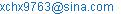(Xu Changxiang)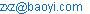(Zhang Xiaozhong)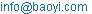(Chen Youjun)

Xu Changxiang, Zhang Xiaozhong, Chen Youjun. Pressure Energy, Resistance and Reactance in Fluid Leak & Flow. Special Issue: Xu’s Sealing and Flowing Theories of Fluids. International Journal of Energy and Power Engineering. Vol. 5, No. 4-1, 2016, pp. 22-30. doi: 10.11648/j.ijepe.s.2016050401.13

Received: June 22, 2016, 2016; Accepted: June 25, 2016, 2016; Published: August 24, 2016

Abstract: The fluid current in a fluid circuit, corresponding to the electrical current in an electrical circuit, is determined by a fluid pressure corresponding to electrical pressure (voltage), and a fluid impedance corresponding to electrical impedance, and directly proportional to the fluid pressure and inversely proportional to the fluid impedance between two ends of the fluid circuit. The fluid impedance is the algebraic sum of the fluid resistance and the fluid reactance between the two ends. Fluid resistance is a physical quantity for measuring the peripheral resistance of a fluid current; fluid reactance, a physical quantity for measuring the front resistance of a fluid current; and leak resistance, a physical quantity for measuring the tightness of a seal. The three quantities have an identical measuring unit, indicating the sustained fluid pressure needed for a unit of fluid currents, or for a unit cubage of fluids for a unit of time, to flow through a fluid resistance, a fluid reactance or a leak resistance, and so (the current) x (the resistance) = (the pressure energy consumed by the resistance), (the current) x (the reactance) = (the pressure energy converted into the kinetic energy by the reactance), (the current) x (the leak resistance) = (the pressure energy consumed by the leak resistance), and (the current) x (the resistance + the reactance) = (the general pressure needed for a fluid current to flow through a fluid circuit). A leak path of seals, almost with kinetic energynegligible, can be considered a typical fluid circuit without any fluid reactance. Reactance of piping is from its each bore-changing passage or port. Reactance from reducing passages or ports is positive, and reactance from enlarging passages or ports is negative. A fluid current flowing past a moving object is equivalent to the one flowing in a pipe's wall-bulged passage whose corresponding right inclusion body has the same axis, generatrix and volume as the object's has. The fluid currents flowing over and under a wing are equivalent to the ones flowing in two parallel contiguous pipe lengths that are placed one under the other and use the length of the wing chord plane as the circumference of their cylindrical inlet and outlet walls, and use the upper and lower average curve surfaces of the wing separately as their upper and lower curve walls. The lift of the wing is from the inner pressure difference of the two pipe lengths.

Keywords: pressure energy, pressure's sustainability, tightness, leak resistance, fluid resistance, fluid reactance

0 Introduction

In comparison, the electronic mass is so tiny that an electrical current of electrons is a flowing body with kinetic energy negligible, and the molecular mass is so huge that a fluid current of molecules is a flowing body with kinetic energy not negligible; i.e. to maintain a unidirectional and steady electronic current in an electrical circuit only needs to consider providing for the circuit a pressure used to overcome an electronic friction or electrical resistance, and to maintain a unidirectional and steady fluid current in a fluid circuit needs to consider providing at the same time for the circuit a pressure used to overcome a peripheral friction of fluid flow and a pressure used to impart a kinetic energy to fluid molecules. The peripheral fraction of a laminar flow in pipes is the fractional resistance from the static fluid adhering to the pipe wall, and the pressure on the fractional resistance is the energy consumed as a fluid flows through the pipe. The pressure used to impart kinetic energy to fluid in a pipe is a kind of energy that can be converted from the kinetic energy back into the pressure without consumption. In other words, an ordinary pipe line, as a fluid flows through it, has both such a pressure drop consuming energy and such a pressure drop not consuming energy that it should correspond to an alternate current circuit with both a resistance consuming energy and a reactance not consuming energy. Accordingly, Poiseuille's law 1 and Darcy's law 2, which correspond to Ohm's law that is suitable for the electrical circuit only with an energy-consuming resistance, are only suitable for the fluid circuit only with an energy-consuming pressure drop as a fluid flows through the circuit, and thus not used for any inner fluid circuit of pipes and any outer fluid circuit of moving objects with kinetic energy not negligible. In other words, the fluid current in ordinary inner and outer fluid circuits, corresponding to the electrical current in an alternate electrical circuit, is determined by a fluid pressure corresponding to electrical pressure, and a fluid impedance corresponding to electrical impedance. However, so far people have not found and defined the fluid reactance and the tightness or leak resistance, which have the same measuring unit as the fluid resistance, and even have incorrectly used the reciprocal of the decompressed fluid current, the volume (converted from the number of liquid drops or gas bubbles) leaking to the atmosphere per unit time, as the measuring unit of tightness 3~4. Therefore, it has to be said that people at present have not well known the flow of fluids.

Both the leak of a pressure vessel and the movement of an object are almost a flow of fluids on a surface of objects. What on earth are the sameness and difference between such fluid flow on objects and the fluid flow in pipes? The present hydromechanics, based on the superficial conclusion (Bernoulli's principle) derived from the principle of conservation of energy and only telling the pressure in a fluid decreases as its velocity increases, even does not know the mechanism and the variable equation of change in pressure with velocity, and thus can only know such an ordinary property of conservation of fluid energy rather than the unique property of fluid energy conversion or fluid mobility as not to uniformly answer or explain these most basic questions about fluid flows.

1 Pressure Energy and Tightness or Leak Resistance

1.1. Xu's Sealing Law and Tightness 

Ten meters of water level difference can just create a pressure of 0.1 MPa (a standard atmospheric pressure) by gravity. Undoubtedly, a general gas or liquid pressure vessel, whose height size is quite limited and whose working pressure is far greater than atmospheric pressure, can be considered to be one with an ignorable influence of its fluid gravity on its pressure, or to be one with a nearly similar static pressure at each inner point. As to such a static pressure vessel, if t is the time expended on leaking all the fluid in it through its sealing joints at a constant pressure p, the product (pt) of pressure p and time t at any allowable constant pressure p is constant and indicates its pressure's sustainability, because the cubage of fluid leaked per unit of time, called a leakage current IL, is directly proportional to the constant pressure p, or because the higher the constant pressure p, the shorter the leak time t, and vice versa.

If leakage occurs under a constant pressure provided by a pressurizing piston, any fluid leaking out of a static pressure vessel is equivalent to the fluid extruded out of the vessel by the piston, and so the energy released from all the pressure fluid leaking out of the vessel shall equal the work W, W = pAl = pC, done by the piston in accordance with the law of conservation of energy. In other words, the pressure energy E stored in the pressure fluid of a static pressure vessel is the product of the pressure p and the cubage C of the vessel, or E = pC, where A = cross-sectional area (m2) of the piton cylinder, l = piston-moved distance (m), p = fluid pressure (N/m2) = energy contained in a unit cubage of fluid (N•m/m3), and C = cubage of the vessel (m3).

If cubage of fluid leaked for a unit of time (C/t) is defined as leakage current (IL), and pressure (p) required to leak a unit cubage of fluid for a unit of time, as leak resistance (RL), or if IL = C/t and RL = p/IL, it can be seen from pressure energy pC = (ptC)/t = ILpt that: (pC)/IL = ptC(p/IL) = ptCRL = pt; i.e. vessel's pressure's sustainability pt identically equals the product of vessel's cubage C and leak resistance RL, or vessel's pressure-sustaining performance is determined by vessel's cubage C and leak resistance RL and can be measured in the length of time (t) expended on leaking all of the vessel's fluid at a constant pressure.

As a summary of the above, it is Xu's sealing law stating that the work (pC/IL) done per unit of leakage current by a pressurizing piston for keeping a pressure vessel or system leaking at a constant leakage current IL or at a constant pressure p is called the pressure's sustainability pt of the vessel or system, whose magnitude is the product of pressure p and time t expended on leaking all the fluid in the vessel or system at a constant pressure p, and also the product of fluid cubage C and leak resistance RL of the vessel or system; i.e. the equation pt = CRL = a constant is tenable for any pressure vessel or system.

If pressure p uses MPa as its unit and leakage current IL uses m3/h as its unit, it can be known in according with Xu's sealing law:

— that the tightness or leak resistance RL (RL = pt/C = p/IL) is the pressure's sustainability pt required for a pressure vessel or system to leak a unit cubage of fluid through its sealing joints under a constant pressure p, equal to the pressure p required for the vessel or system to leak per unit of leakage current IL or leak a unit cubage of fluid per unit time under a constant pressure, whose unit is Xu = MPa•h•m-3; and

— that the leak conductance LR (LR =1/RL) is the reciprocal of leak resistance, equal to the cubage of fluid leaked out of a pressure vessel or system through its sealing joints per unit expenditure of pressure's sustainability, whose unit is Ux = m3(MPa•h)-1;

i.e. the tightness or leak resistance RL of a pressure vessel is the pressure's sustainability pt of a unit cubage of the pressure vessel, dependent on neither its cubage nor its test pressure in magnitude, and so can be by no means identified by using either a cubage-dependent pressure duration or a pressure-dependent leakage current leaked outside that is arbitrarily prescribed in the prior ISO standards 3~4.

If a pressure vessel leaks a unit cubage of fluid per unit of time under pressure p (Fig.1b), then it needs two units of time for it to leak a unit cubage of fluid under pressure 0.5p (Fig.1a), and it needs half a unit of time for it to leak a unit cubage of fluid under pressure 2p (Fig.1c), for the leakage current or the cubage of fluid leaked per unit of time decreases by half or doubles as the pressure decreases by half or doubles. In other words, it can be seen from Fig.1 that the tightness or leak resistance RL of any pressure vessel equals the product of pressure p and time t expended on leaking a unit cubage of fluid or RL = pt/C, not changing with its cubage and pressure. However, the pressure energy lost by leaking a unit cubage of fluid out of a pressure vessel changes with its pressure, and the lower the pressure, the less the lost energy, and vice versa. For example, the lost energy in Figs.1a/1b/1c is separately 0.5p/p/2p. Therefore, it is very worth attention that the release pressure of tap water and discharging systems closely relates to energy conservation and emission reduction.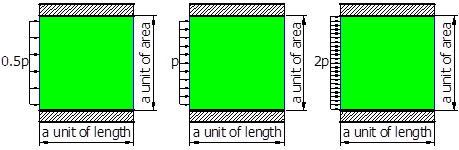(a) t = two units        (b) t = one unit       (c) t = half a unit

Fig. 1. Diagrammatic illustrations of Xu's sealing law

1.2. Gauging of Tightness or Leak Resistance 

It can be seen from Xu's sealing law that the tightness or leak resistance RL of a pressure vessel or system is equal to pΔt/ΔC. Hence, a test vessel can be used to gauge the tightness of its seals according to its cubage ΔC of fluid leaked for a time Δt at a constant pressure p; i.e. the cores of a tightness-gauging device are first to provide a constant test pressure p and then to record or to gauge the cubage ΔC of fluid leaked out of a tested vessel for time Δt under the pressure p.

As shown in Figs.2~4, the constant test pressure p is provided to a test port by a piston cylinder (04), a piston (05), weights (06) and passage parts (03). With leaking through sealing joints, the piston will descend and the product of piston-descended height and cylinder's cross-sectional area is the cubage ΔC of fluid leaking out of a tested vessel or system at a constant pressure p. Accordingly, ΔC and Δt needed to calculate or ascertain the total leak resistance of the tested vessel or system can be obtained by a recorder of change in height with time that can have the height gauge (07) and the timer (08) set to zero at the same time by a button. For a certain accuracy of the test pressure and the leaked cubage, the axes of the weight, the piston and the cylinder shall be coaxially perpendicular to the level (01) of the height gauge. Fig.2 shows a device for gauging or verifying the piston's leak resistance; Fig.3, for gauging the leak resistance of cylinder's joints; and Fig.4, for gauging the total leak resistance of the constant pressure supply including the piston, the cylinder and the passage parts. The total leak conductance (the reciprocal of total leak resistance) of the constant pressure supply equals the sum of each leak conductance components of the piston, the cylinder and the passage parts. If the total leak resistance of the pressure supply can be infinite relative to the leak resistance of a tested vessel or system, it needs no medium calculation of leak conductance to gauge the leak resistance of the tested vessel or system.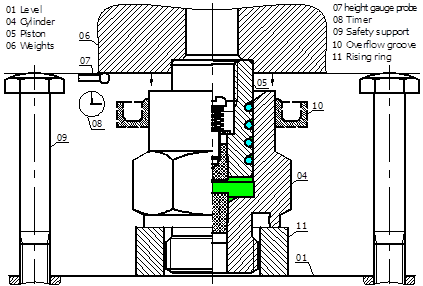Fig. 2. Gauging the piston's leak resistance.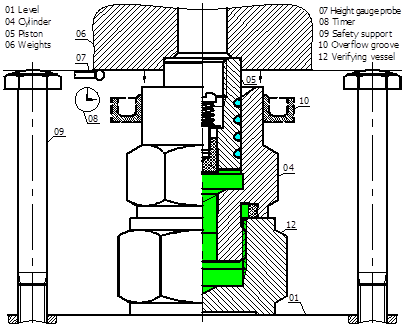Fig. 3 Gauging the cylinder's leak resistance.

1.3. Xu's Sealing Theorem and Pressure's Sustainability Gauge 

Xu's sealing theorem states that any fluid leakage out of a static pressure vessel or system will cause its pressure to decay; if Δt is the time expended on the leakage-caused pressure decay of a pressure vessel or system from pressure p to (pΔp), its pressure's sustainability pt = p(p – 0.5Δp)Δt/Δp becausep/Δp is the total number of subvessels of the vessel or system and (p – 0.5Δp)Δt is the pressure's sustainability pt of a subvessel of the vessel or system under a constant pressure (p – 0.5Δp) when its leakage is gauged according to pressure decay of Δp; and obviously the greater the value of p/Δp, the shorter the time needed to observe or gauge leakage, the closer to gauging under a constant pressure and a constant temperature, the more accurate the gauged value, and the more capable of substituting p for (p0.5Δp) in the pt-calculating expression.

It can be seen from Xu's sealing theorem that the pressure's sustainability pt of a pressure vessel or system is equal to p(p – 0.5Δp)Δt/Δp. Hence, a test vessel with a known cubage C can be used to gauge the tightness or leak resistance RL of its seals, RL = p(p – 0.5Δp)Δt/(ΔpC), according to its pressure decay caused by fluid leakage from a pressure p to (pΔp) for a time Δt. Because the pressure's sustainability pt (pt = CRL) of a vessel or system is determined by its fixed cubage C and leak resistance RL, it can quickly gauge the state of its seals and can calculate the distance from the leaking point to have pressure's sustainability gauges fixed at its one or two ends for recording its pressure decay Δp and the time Δt expended on the pressure decay under the condition that it is isolated from the pressure supply for a certain time, thus being capable of avoiding macroscopic observation of its leakage in a narrow room and in a long pipeline.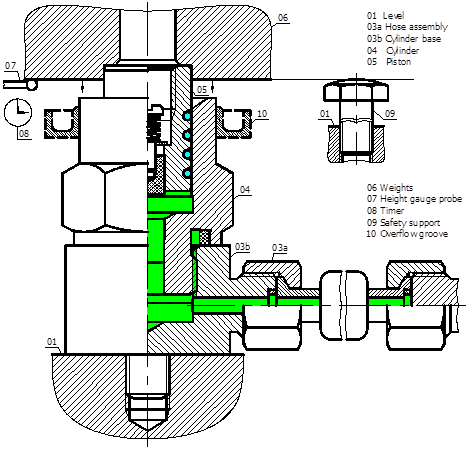Fig. 4. Gauging the leak resistance of any pressure vessel and system.

2 Pressure Energy and Fluid Resistance and Reactance

2.1. Pressure Energy and Fluid Resistance and Reactance of Pipes

Newton proposed that there is an interacting friction between two adjacent laminas of flowing fluids 6. Coulomb, using an uncoated disk, a wax-coated disk and a sand-coated disk hung in liquid and having an identical initial turn angle for their free oscillation experiments, verified that their attenuations have nothing to do with their surface roughness and only relate to the viscosity of the liquid, or that the frictional resistance of a liquid to an oscillating disk is from the friction between the liquid adhering to the disk and the liquid flowing past the disk 6. In other words, the frictional resistance to a fluid flowing in pipes is from the friction between the static liquid adhering to the pipe wall and the flowing fluid, not directly from the friction between the pipe wall and the liquid. Poiseuille proved that the frictional resistance of a fluid flowing in tubes RF = (8ηl)/(πr4) (where η = viscosity, l = tube length, r = tube wall radius), which not only has nothing to do with the tube wall roughness but also is not measured in Newton and is the ratio of the pressure p to the fluid current IF between two tube ends, or fluid resistance RF = p/IF and corresponds to electrical resistance 1.

The unit of fluid velocity [m/s] = [(m3/m2)/s], and so the fluid velocity v = the fluid cubage flowing through a unit cross-section per unit time. Therefore, the fluid current (IF) defined as the fluid cubage flowing through a cross-section per unit time equals the product of the cross-sectional area (A) and the velocity (v), or the fluid current IF = Av and can be construed as the volume of a fluid cross-section with an area A moving at a velocity v for a unit of time.

Theoretically, the kinetic energy of a fluid results from its pressure doing work to its static body, or in a flowing fluid its kinetic energy 0.5mv2 = pAl → 0.5ρv2 = p, where m = fluid mass, v = fluid velocity, ρ = m/(Al) = fluid density, p = pressure that did work to a static fluid or the pressure that was used to give the fluid the kinetic energy and does not exist in the fluid flowing at the said velocity v, and l = distance through which the fluid cross-section with area A is moved by force pA. Therefore, any fluid has a conversion of pressure into kinetic energy as it is from its rest to its flow, a conversion of kinetic energy into pressure as it is from its flow to its rest, and an unceasing mutual conversion of pressure and kinetic energy in its velocity-changing flow, always having a pressure decrement or increment equal to a kinetic energy increment or decrement in a unit cubage of fluid.

It is because the pressure's unit Pa = N/m2 = N•m/m3 = the energy stored in a unit cubage of fluid and the kinetic energy in a unit cubage of fluid is 0.5ρv2 that the mutual conversion of pressure and kinetic energy in a fluid is a formal conversion of two kinds of equivalent energy contained in the fluid, and a speed increase and a pressure decrease arise at the same time as the pressure (energy) is converted into the kinetic energy, and vice versa.

From the equation that the kinetic energy per unit cubage of fluid = 0.5ρv2 = p = the pressure used to impart the kinetic energy to the fluid, it can be seen that: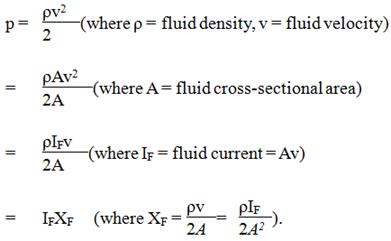The quantity XF, XF = p/IF, not only can have the same measuring unit Xu (MPa•h•m-3) as fluid resistance and leak resistance have but also is a factor causing pressure (energy) to be converted into kinetic energy of fluid without any energy loss. Therefore, according to electric reactance, the quantity XF should be called fluid reactance of a fluid circuit, and the product (IFXF) of the fluid reactance (XF) and the fluid current (IF), called a pressure drop caused by the fluid reactance.

From XF = p/IF = (ρv)/(2A), it can be seen that fluid reactance (XF) is the work (p) done by the pressure (p) needed by a flow of one unit of fluid current (IF = 1) or one unit cubage of fluid per unit of time (IF = C/t =1). Accordingly, as to a unit cubage of fluid (ρ), the smaller the cross-sectional area of fluid, the longer the length of the fluid, and of course the greater the work (XF) done by an identical pressure (p).

Because pipe's reactance XF = (ρv)/(2A) or is changed with pipe's inner cross-sectional area A, the pressure drop IFXF caused by pipe's reactance is leaped with pipe's inner cross- sectional area A in the flowing direction, or the reactance- caused conversion of pressure into kinetic energy is instant, or the conversion of pressure into kinetic energy results from a reactance-caused transient strike of a pressure p on fluid, or any fluid, on flowing, has devoured a strike pressure causing it to flow. Because a fluid flow also needs another pressure used to overcome the friction, it needs two pressure components in total. One is the pressure IFXF for imparting the flow to fluid, and one, the pressure IFRF for overcoming the flowed friction of fluid; i.e. the total pressure drop p of a fluid flow in a pipe is the sum of the two pressure drops of IFRF and IFXF, or p = IFRF + IFXF = IF(RF + XF), or any pipe line is a series fluid circuit of its fluid resistance RF, RF = (8ηl)/(πr4), and its fluid reactance XF, XF = (ρv)/(2A).

Pipe's fluid resistance is the frictional resistance of the static liquid adhering to the pipe wall to the periphery of the flowing fluid. Because pipe's fluid resistance RF = p/IF = p/(C/t) = (pt)/C, it can be construed either, in accordance with RF = (pt)/C, as the product of pressure and time or the pressure's sustainability pt needed for a unit cubage (C) of fluid flowing through pipes to overcome its peripheral friction, or, in accordance with RF = p/IF, as the pressure p needed for a unit of fluid current (IF) flowing through pipes or for a unit cubage (C) of fluid flowing through pipes per unit of time (t) to overcome its peripheral friction.

Since the kinetic energy possessed by a body theoretically results from a force doing work to its static body and equals the product of the force and the distance through which the  force moves the body in the direction of the force, the positive or negative active forces causing a piped fluid to have an increment or decrement of kinetic energy are both in the axial direction of the pipe; i.e. the fluid reactance resulting in a mutual conversion of pressure and kinetic energy in a piped fluid is the axial or frontal positive or negative resistance from bore-changing passages or ports of pipes to fluid, and the reactance from reducing passages or ports is positive and from enlarging passages or ports, negative. Because pipe's reactance XF = p/IF = p/(C/t) = (pt)/C, it can be construed either, in accordance with RF = (pt)/C, as the product of pressure and time or the pressure's sustainability pt needed for a unit cubage (C) of fluid flowing past bore-changing passages or ports to avoid its frontal resistance, or, in accordance with RF = p/IF, as the pressure p needed for a unit of fluid current (IF) flowing past bore-changing passages or ports or for a unit cubage (C) of fluid flowing through bore-changing passages or ports per unit time (t) to avoid its frontal resistance.

As shown in Figs.5a and 5a', the fluid reactance of the windward (a) piping the fluid into the wall-bulged passage (c) and causing some pressure energy to be converted into kinetic energy in the piped fluid is positive, and the fluid reactance of the leeward (a') piping the fluid out of the wall-bulged passage (c) and causing some kinetic energy to be converted into pressure energy in the piped fluid is negative. Because the fluid reactance XF,XF= (ρIF/2)(1/Ab2–1/Aa2), of a reducing passage (a) is determined by its major and minor cross-sectional areas Aa and Ab, the windward and the leeward that are disposed one after the other and whose major and minor diameters are separately the same always have such a reactance and such a pressure drop or rise equal in magnitude and opposite in direction as to cancel each other out, and even a different shape of the windward or leeward at most causes their equal pressure drop or rise a different pressure gradient. However, the fluid resistance of the windward and leeward cannot cancel each other out, and the total resistance of the wall-bulged passage (c) is the sum of each resistance of its windward (a), leeward (a') and straight (b) passages, where the resistance of the reducer and enlarger can be calculated according to the mean radius of their major and minor ends.

A pipe line is merely made up of some reducing, enlarging and straight passages. Accordingly, its total impedance can be calculated in accordance with each calculating formula for their fluid resistance and reactance, and then the supply pressure can be seen at a glance needed to ensure a pipe line without any leakage a required fluid current.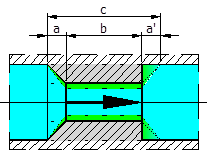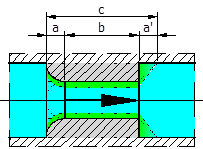(a) Pipe's wall-bulged passages with a conical windward surface (a') Pipe's wall-bulged passages with an arc windward surface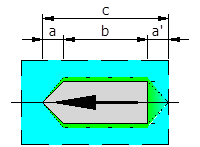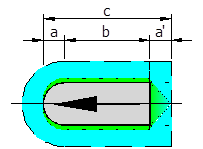(b) Moving cylindrical objects with a tapered windward surface (b') Moving cylindrical objects with a spherical windward surface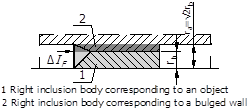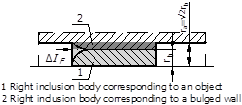(c) The bulged wall and the cylindrical object whose corresponding right inclusion bodies have the same volume (c') The bulged wall and the cylindrical object whose     corresponding right inclusion bodies have the same volume

Fig. 5. Pipe's wall-bulged passages corresponding to axially moving cylindrical objects.

2.2. Pressure Energy and Fluid Resistance and Reactance of Moving Objects

2.2.1. Pressure Energy and Fluid Resistance and Reactance of Axially Moving Cylindrical Objects

If an axial movement of a cylindrical object in the atmosphere, the river, the lake and the sea does not change the free inherent pressure around it, the movement is fully equivalent to a movement of the object in a certainly pressurized pipe and the free inherent pressure region is a radial wall and an axial certain pressure of the pipe because the free region only has some axial pressure difference but not any radial pressure difference relative to the moving object and can cause only an axial flow rather than any radial flow of fluids.

Movements are relative. The rapid flow of a fluid through a wall-bulged passage of certainly pressurized pipes can be regarded as a movement of the bulged wall in a static fluid at a certain pressure. The axial movement of a cylindrical object in the atmosphere, the river, the lake and the sea can be regarded not only as the object's movement in a certainly pressurized pipe but also as the rapid flow of a fluid in a certainly pressurized pipe past a static object.

The volume of fluid moved separately by a cylindrical object and a pipe's wall-bulged passage as they each axially move a body length in a static fluid at a certain pressure is equal to the volume of each corresponding right inclusion body whose cross-section is each largest cross-section and whose length is each total length, and thus the axial movement of both a cylindrical object and a pipe's bulged wall in a static fluid at a certain pressure is to cause some static fluid whose cubage equals the volume of their each corresponding right inclusion body to flow between each surface and the static fluid, and not to influence the axial movement of the static fluid in the original position relative to the object and the wall.

Concretely, the flowing of a fluid past a wall-bulged passage of pipes (Figs.5a and 5a') can be regarded as a movement of the bulged wall in a static fluid at a certain pressure, which causes a fluid current increment ΔIF, ΔIF = A (the largest cross-sectional area of the bulged wall) x v (fluid velocity), to hustle between the bulged wall and the static fluid; whereas the axial movement of a cylindrical object (Figs.5b and 5b') in the atmosphere, river, lake and sea can be regarded as a flow of a fluid in a pipe formed by the free region past a static object, which also causes a fluid current increment ΔIF, ΔIF = A (the largest cross-sectional area of the object) x v (object velocity), to hustle between the object and the static fluid. If the right inclusion bodies corresponding to the object and the bulged wall share an axis, a generatrix and a radius equation of ra = 20.5rb (Figs.5c and 5c'), their movements relative to fluid are the movement of two right inclusion bodies with the same volume and length relative to a static fluid at a certain pressure, and both cause a fluid current with a similar increment ΔIF (ΔIF = πrb2v) and a similar total IF (IF = πra2v = 2πrb2v) to flow past a static object or a pipe's wall-bulged passage with an identical fluid interface. Thus the fluid current, the fluid resistance, the fluid reactance, the fluid impedance and the required power of an axially moving cylindrical object at a certain velocity can be calculated according to each formula of a pipe's wall-bulged passage.

Rockets, missiles, airplane bodies and submarines are of an ordinary cylindrical vehicle moving in axial direction. The basic requirements for them are merely capable of having a large loading space and a small fluid resistance. As to vehicles with an identical loading space and moving speed, their fluid resistance RF, RF = (8ηl)/(πr4), decreases as the square of their body's major cross-sectional area increases, and increases as their body length increases. Therefore, in order to have a larger loading space and a smaller fluid resistance, they shall have a nearly right cylindrical body with a cross-sectional area as larger as possible and with windward and leeward passages as shorter as possible. However, such a right cylindrical body requires a greater start power Ap to reach a required speed.

2.2.2. Pressure Energy and Fluid Resistance and Reactance of Wings

A wing is an unrolled object of two parallel contiguous pipe lengths placed one under the other. The chord and the upper and lower median curves of a wing's cross-sectional profile separately form the longitudinal section profiles of the two upper and lower pipe lengths. The length of wing chord plane is the circumferences of inlet and outlet cylinders of the two pipe lengths. The two pipe lengths have the same inlet and outlet bores for air and thus the same fluid currents, but have such a different longitudinal section profile and thus have such a different axial fluid resistance and reactance distribution as to have a different axial pressure distribution. The lift of wings results from the inner pressure difference of the two upper and lower pipe lengths.

As for a half-cambered wing (Fig.6), whose upper surface is cambered and whose chord plane is its lower surface, it is equivalent to two parallel contiguous pipe lengths whose upper length is one wall-bulged passage of pipes with a windward followed by a leeward and whose lower length is one straight passage of pipes, its mean greatest camber (h) being the greatest wall thickness of the upper pipe length and its length being the circumference of inlet and outlet cylinders of the upper and lower pipe lengths. Because the upper and lower pipe lengths have an identical inlet and outlet bores (2ra), they have an identical fluid current IF = πra2v, where v is the velocity of the wing relative to the free atmosphere region. However, because the upper pipe length has a smaller average bore (2r) or a smaller average inner section area (A = πr2) than the lower pipe length, the former has a greater fluid resistance RF = (8ηl)/(πr4) and a greater fluid reactance XF = (ρv)/(2A) and thus a greater pressure drop of IFRF and IFXF than the latter, i.e. the pressure in the upper pipe length is more negative than in the lower pipe length, which results in a wing having a certain lift.

An airplane will keep in horizontal flight (Fig.6a) at a constant speed when wing's velocity causes wing's lift to equal airplane's gravity. An airplane will have anascending component in flight when wing's velocity causes wing's lift to be more than airplane's gravity.An airplane will have a descending component in flight when wing's velocity causes wing's lift to be less than airplane's gravity. Because wing's front respectively has an ascending and a descending increment greater than wing's back when in accelerating and decelerating flights, an airplane always keeps its head upward in ascending flight (Fig.6b), and keeps its head downward in descending flight. When flight speed and air density have no too great change, the airplane's horizontal (Fig.6a), ascending (Fig.6b) and descending flights are almost equivalent to a similar compressive air flowing in different orientation of pipes in a level plane and hardly have no change in the ratios of fluid currents, fluid resistances and fluid reactances on/of wing's upper and lower surfaces or hardly have no change in the ratio of fluid pressures over and under the wing, but have a change in the level actuation area of ascending pressure on the wing and thus in wing's lift. Airplane's take-off is such a changing flight from its horizontal movement to its heading-upward oblique flight as to cause a decreasing level actuation area of ascending pressureon its wing, while airplane's landing is such a changing flight from its heading-downward oblique flight to its horizontal movement as to cause an increasing level actuation area of ascending pressure on its wing. Thus an airplane has a decreasing lift and needs an acceleration as it is leaving the ground, but has an increasing lift and needs a deceleration as it is landing. It is also not difficult to understand an airplane has a lift increment just before it lands.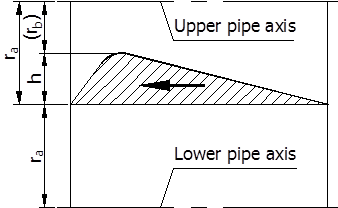(a) Wings in horizontal flight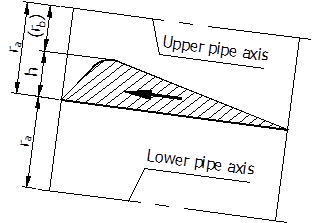(b) Wings in ascending flight

Fig.6 Upper and lower pipe lengths corresponding to a wing

Therefore, airplane's lift and power for flight can be calculated according to the fluid current, resistance and reactance in two corresponding upper and lower pipe lengths.

3 Influence of Turbulent Flow on Fluid Resistance and Reactance

Dust on solids in low speed water always keeps not being flushed out, but a river-bed can be flushed out by high speed flood. The two facts explicitly illustrate that any low speed water has no friction with the solid surface passed by the water and results in fluid resistance having nothing to do with surface roughness, and that high speed water has friction with solid surface passed by the water and results in fluid resistance having something to do with surface roughness. The limit for fluid resistance to have nothing and something to do with surface roughness is dependent on if the surface has a fluid adhered lamina capable of resisting fluid scour.

When a pipe wall has such a fluid adhered lamina capable of resisting fluid scour, the wall can produce a peripheral friction and a radial reaction force to passing fluid only by the smooth fluid adhered lamina rather than by wall's micro-rises. The peripheral friction is circumferentially symmetrical and only has something to do with fluid viscosity. The radial reaction force is also so circumferentially symmetrical that the fluid in circular pipe walls has no radial flowing power and can only flow in laminar flow along pipe axis.

When a pipe wall has no fluid adhered lamina capable of resisting fluid scour, the fluid flowing past the wall will be randomly reflected by some wall's micro-rises that are so uncertain in orientation, angle, and magnitude as to cause an axial backflow and a radial flow or a turbulent flow that is asymmetric in both magnitude and deepness, and thus cause the fluid resistance and reactance calculating formulas based on laminar flows to lose correctness.

Fluid viscosity determines the ability for an adhered fluid to preventa pipe wall from being soured out, and fluid inertia determines the ability for a flowing fluid to cause a pipe wall to be scoured out. When the fluid adhered lamina can fully resist fluid scour, the pipe wall will not have any such direct scour from the fluid passing it as to ensure the fluid a laminar flow in the pipe. When the fluid adhered lamina cannot fully resist fluid scour, the pipe wall will have some such direct scour from the fluid passing it as to cause the fluid a turbulent flow in the pipe. When the scour-resisting ability of a fluid adhered lamina roughly matches the scouring ability of a flowing fluid, the fluid current will be a transitional current between the laminar flow and the turbulent flow. Because a Reynolds number is the ratio of the inertia force to the viscous force of a fluid, circular pipe walls with different surface roughness and different surface shape symmetry have a different fluid inertia-reflecting ability or a different critical Reynolds number of laminar flow, or the values of calculated fluid resistance and reactance of a pipe shall be appropriately corrected in accordance with Reynolds number associated with surface roughness and surface shape symmetry, or a pipe with a wall smoother than an ordinary wall shall have a Reynolds number greater than the ordinary critical Reynolds number or have a higher velocity limit for calculating its fluid resistance and reactance according to laminar flow.

Water drops, over the years, can scour out a hole in stone but there is no scouring erosion on windward of pipe lines and vehicles such as automobiles and ships. The two phenomena explicitly illustrate that the scour effects of steady and unsteady currents to their axial ends are different from each other, or that a steady current can automatically form such a bore-changing passage in front of or behind its axial end as to have no turbulence influencing the correctness of calculated fluid resistance and reactance in general.

4 Conclusions

Though the kinetic energy 0.5ρv2 possessed per unit cubage of fluid was early called a dynamic pressure, it has been unknown that the kinetic pressure 0.5ρv2 is equal to the product of fluid current IF and fluid reactance XF in a fluid circuit 7. Poiseuille proved that the fluid current IF through a capillary is directly proportional to the pressure difference Δp between its two ends and the constant of proportionality is the fluid resistance RF, or it has been known that the pressure drop Δp = IFRF when a fluid current flows through a capillary, but it has been unknown why it is not suitable for an ordinary pipe. In other words, the above facts have proved that, without concepts of fluid resistance and reactance, it is impossible to know that the fluid current through a fluid circuit is directly proportional to the pressure difference between its two ends, or have proved that Bernoulli's principle or equation only reveals an ordinary property of conservation of fluid energy rather than the unique property of fluid mobility. In fact, it is only after it is realized both that fluid resistance and reactance have the same measuring unit and that leak paths are a fluid circuit with fluid kinetic energy or reactance negligible that it can be realized that an ordinary pipe line has both fluid resistance and fluid reactance. It is people’s ignorance of the fact that an ordinary pipe has both fluid resistance and fluid reactance that results in people having thought that Poiseuille's law is only applicable to a very thin tube rather than an ordinary pipe 8, and also results in fluid resistance having not been defined and used according to Poiseuille's law.

Therefore, after having the definition of leak resistance after Xu's sealing law, people not only have means to quantize and test the tightness used to prove Xu's seal-designing theory 9~10 but also have two derived quantities of fluid resistance and reactance required to describe uniformly various flows of fluid in leak paths and pipe lines and on moving objects. Meanwhile, Xu's sealing law is again further proved by the fact that various problems of fluid flows are uniformly solved under the concepts of fluid resistance and reactance.

Note: This paper was initially published in Chinese magazine of Hydraulics Pneumatics & Seals, 2015, Volume 35 (9): 4-10.

References

1. Hagen-Poiseuille equation. TheFree Encyclopedia, Wikipedia.http://en.wikipedia.org/wiki/Hagen–Poiseuille_equation
2. Darcy's law.TheFree Encyclopedia, Wikipedia.http://en.wikipedia.org/wiki/Darcy's_law
3. ISO 19879-2005,Metallic tube connections for fluid power and general use —Test methods for hydraulic fluid power connections [S]
4. ISO 5208-2008, Industrial valves — Pressure testing of metallic valves [S]
5. XU Changxiang.Definition, Quantifying and Gauging of Tightness. Petro-Chemical Equipment, 2014, 43(3):11-14
6. DING Zurong. Fluid Mechanics: Volume I [M]. Beijing: Higher Education Press, 2004)
7. Bernoulli's principle.TheFree Encyclopedia, Wikipedia. http://en.wikipedia.org/wiki/Bernoulli%27s_principle
8. Poiseuille's Law. The Great Soviet Encyclopedia, 3rd Edition. http://encyclopedia2.thefreedictionary.com/Poiseuille's+law
9. XU Changxiang. XU's Sealing Theory and Rectangular & O-Shaped Ring Seals [J]. Petro-Chemical Equipment, 2013, 42(2): 78-85.
10. XU Changxiang. XU's Designs and Parameters for Sealing Elements [J]. Petro-Chemical Equipment, 2013, 42(3): 58-63.

 Contents 0 1 1.1. 1.2. 1.3. 2 2.1. 2.2. 3 4
Article ToolsAbstractPDF(1614K)